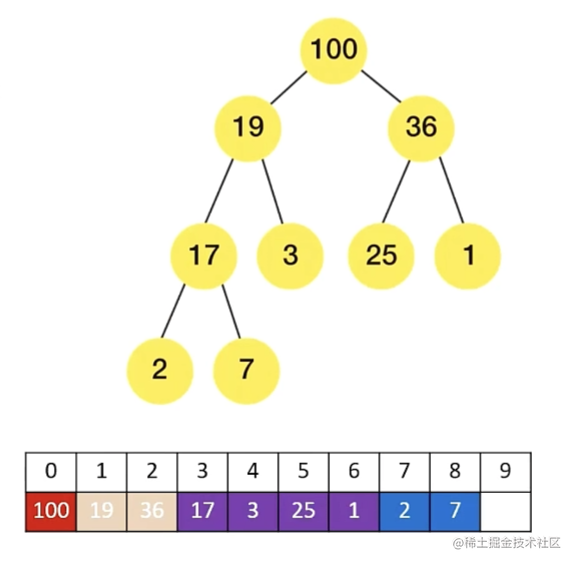2023-02-2300

## 目录

1. 认识堆结构
2.堆的应用
3. 堆结构的设计
4. 堆结构的封装
4.1 基础框架 v1 版
4.2 添加 insert 方法 v2 版
4.3 添加 extract 方法 v3 版
4.4 添加 buildHeap 方法 v4 版

# 1. 认识堆结构

1. 堆是一种特殊的完全二叉树
2. 所有的节点都大于等于（大顶堆）小于等于（小顶堆） 他的子节点
3. `js` 中通常使用数组表示堆
1. 左侧子节点的位置 `2 * index + 1`
2. 右侧子节点的位置 `2 * index + 2`
3. 父节点的位置 `（index - 1）/ 2`# 2.堆的应用

1. 堆能快速高效的找出最大值和最小值 `O（1）`
2. 找出第 `k`最大（小）元素
1. 构建一个最小堆，并将元素依次插入堆中
2. 当堆的容量超过 `K`，就删除堆顶
3. 插入结束后，堆顶就是第 `K` 个最大元素

# 3. 堆结构的设计

1. `data`：存储堆结构的元素，通常使用数组来实现
2. `size`：堆中当前元素的数量

1. `insert(value)`：在堆中插入一个新元素
2. `extract/delete()`：从堆中删除最大/最小元素
3. `peek()`：返回堆中的最大/最小元素
4. `isEmpty()`：判断堆是否为空
5. `buildHeap(list)`：通过一个列表来构造堆

# 4. 堆结构的封装

## 4.1 基础框架 v1 版

```ts```class Heap<T> {
// 属性
data: T[] = [];
private length: number = 0;

// 私有工具方法
private swap(i: number, j: number) {
[this.data[i], this.data[j]] = [this.data[j], this.data[i]];
}

insert(value: T) {}

extract(): T | undefined {
return undefined;
}

peek(): T | undefined {
return this.data;
}

size() {
return this.length;
}

isEmpty() {
return this.length === 0;
}

buildHeap(arr: T[]) {}
}
``````

## 4.2 添加 insert 方法 v2 版

1. 因为每次插入元素后，需要对堆进行重构，以维护最大堆的性质
2. 这种策略叫上滤
``````// 方法
insert(value: T) {
// 1. 将元素放到数组的尾部
this.data.push(value);
this.length++;

// 2. 维护最大堆的特性（最后位置的元素需要进行上滤操作）
this.heapify_up();
}

private heapify_up() {
let index = this.length - 1;
let parentIndex = Math.floor((index - 1) / 2);
while (index > 0) {
if (this.data[index] <= this.data[parentIndex]) {
break;
}

this.swap(index, parentIndex);

index = parentIndex;
}
}
``````

## 4.3 添加 extract 方法 v3 版

1. 因为你每次删除元素后，需要对堆进行重构，以维护最大堆的特性
2. 这种向下替换元素的策略叫做下滤
```ts```private heapify_down(index: number) {
while (2 * index + 1 < this.length) {
// 1. 找到左右子节点
let leftChildIndex = 2 * index + 1;
let rightChildIndex = leftChildIndex + 1;

// 2. 找到左右子节点节点较大的值
let largeIndex = leftChildIndex;
if (rightChildIndex < this.length && this.data[rightChildIndex] > this.data[leftChildIndex]) {
largeIndex = rightChildIndex;
}
// 3. 较大的值和 index 位置进行比较
if (this.data[index] >= this.data[largeIndex]) {
break;
}
// 4. 交换位置
this.swap(index, largeIndex);
index = largeIndex;
}
}

extract(): T | undefined {
// 1. 判断元素的个数为 0 或者 1 的情况
if (this.length === 0) return undefined;
if (this.length === 1) {
this.length--;
return this.data.pop()!;
}

// 2. 提取并且需要返回的最大值
const topValue = this.data;
this.data = this.data.pop()!;
this.length--;

// 3. 维护最大堆的特性：下滤操作
this.heapify_down(0);

}
``````

## 4.4 添加 buildHeap 方法 v4 版

`buildHeap` 方法用于原地建堆，即指在建立堆的过程中，不使用额外的内存空间，直接在原有数组还是那个进行操作。

```ts```constructor(arr: T[]) {
this.buildHeap(arr)
}

buildHeap(arr: T[]) {
this.data = arr;
this.length = arr.length;

let start = Math.floor((this.length - 1) / 2);
for (let i = start; i >= 0; i--) {
this.heapify_down(i);
}
}
``````